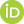## Publication Details

 Category Text Publication Reference Category Journals DOI 10.1002/nme.1770 Title (Primary) Object-oriented finite element analysis of thermo-hydro-mechanical (THM) problems in porous media Author Wang, W.; Kolditz, O.Journal International Journal for Numerical Methods in Engineering Year 2007 Department ENVINF Volume 69 Issue 1 Page From 162 Page To 201 Language englisch Keywords object-oriented programming; finite element method; multi-field problems; porous media Abstract The design, implementation and application of a concept for object-oriented in finite element analysis of multi-field problems is presented in this paper. The basic idea of this concept is that the underlying governing equations of porous media mechanics can be classified into different types of partial differential equations (PDEs). In principle, similar types of PDEs for diverse physical problems differ only in material coefficients. Local element matrices and vectors arising from the finite element discretization of the PDEs are categorized into several types, regardless of which physical problem they belong to (i.e. fluid flow, mass and heat transport or deformation processes). Element (ELE) objects are introduced to carry out the local assembly of the algebraic equations. The object-orientation includes a strict encapsulation of geometrical (GEO), topological (MSH), process-related (FEM) data and methods of element objects. Geometric entities of an element such as nodes, edges, faces and neighbours are abstracted into corresponding geometric element objects (ELE-GEO). The relationships among these geometric entities form the topology of element meshes (ELE-MSH). Finite element objects (ELE-FEM) are presented for the local element calculations, in which each classification type of the matrices and vectors is computed by a unique function. These element functions are able to deal with different element types (lines, triangles, quadrilaterals, tetrahedra, prisms, hexahedra) by automatically choosing the related element interpolation functions. For each process of a multi-field problem, only a single instance of the finite element object is required. The element objects provide a flexible coding environment for multi-field problems with different element types. Here, the C++ implementations of the objects are given and described in detail. The efficiency of the new element objects is demonstrated by several test cases dealing with then no-hydro-mechanical (THM) coupled problems for geotechnical applications. Copyright (c) 2006 John Wiley & Sons, Ltd Persistent UFZ Identifier https://www.ufz.de/index.php?en=20939&ufzPublicationIdentifier=2403 Wang, W., Kolditz, O. (2007): Object-oriented finite element analysis of thermo-hydro-mechanical (THM) problems in porous media Int. J. Numer. Methods Eng. 69 (1), 162 - 201